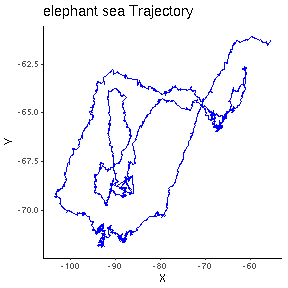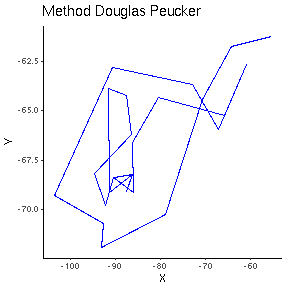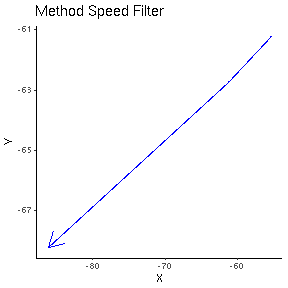## TrajDataMining

The TrajDataMining contains a set of methods for trajectory data preparation, such as filtering, compressing and clustering, and for trajectory pattern discovery. The meth- ods for data preparation are important to prepare trajectory data sets before the data mining phase. TrajDataMining contains the following methods:

• A speed filter that filters out trajectory observations whose speeds are above a user-defined maximum velocity ( ETIENNE , 2011).

• Two compression algorithms: (1) Douglas-Peucker which reduces trajectories by preserving spatial precisions ( DOUGLAS; PEUCKER , 1973), and (2) Open-window Meratnia-By which reduces trajectories by preserving spatiotempo- ral precisions ( MERATNIA; BY , 2004).

• Two algorithms to discover when objects stop and move, CB-SMoT ( PALMA et al. , 2008) and DB-SMoT ( ROCHA et al. , 2010), which can be used to semanti- cally enrich the trajectory data.

• A method, named Partner, that identifies objects that are moving together. We propose this method to recognize trajectories that stay together, based on trajectory distance time series analysis.

## Example data gives

``````library(TrajDataMining)
library(ggplot2)
library(sp)
library(magrittr)
suppressMessages(library(rgdal))
``````

## Plot of A1 data example

### A1 track its a trajectory of sea elephant

``````  df <- data.frame(x=A1@sp@coords[,1],y=A1@sp@coords[,2])

ggplot(df,aes(x=df\$x,y=df\$y))+geom_path(aes(group = 1), arrow = arrow(),color='blue')+ggtitle("elephant sea Trajectory")+xlab("X")+ ylab("Y")+theme_classic()
``````## Douglas Peucker Method

### Method that reduces a trajectory spatially

``````# get the maximum distance
max <- max(A1@connections\$distance)

douglasp <- douglasPeucker(A1,max)

df <- data.frame(x=douglasp@sp@coords[,1],y=douglasp@sp@coords[,2])
ggplot(df,aes(x=df\$x,y=df\$y))+geom_path(aes(group = 1), arrow = arrow(),color='blue')+ggtitle("Method Douglas Peucker")+xlab("X")+ ylab("Y")+theme_classic()
``````## Speed Filter

### Method removes regions where speed was higher than the defined parameter

``````# get the maximum distance
sf <- speedFilter(A1,0.01)
``````
``````##  "2008-01-23 21:32:44"
##  "2008-01-24 01:31:26"
##  "2008-01-24 03:12:00"
##  "2008-01-24 11:24:42"
##  "2008-07-21 04:30:30"
##  "2008-07-21 11:22:30"
##  "2008-07-22 01:09:49"
##  "2008-07-25 13:19:46"
##  "2008-07-28 11:40:23"
##  "2008-07-29 01:23:43"
##  "2008-07-31 09:15:41"
##  "2008-11-19 12:38:34"
``````
``````  df <- data.frame(x=sf@sp@coords[,1],y=sf@sp@coords[,2])

ggplot(df,aes(x=df\$x,y=df\$y))+geom_path(aes(group = 1), arrow = arrow(),color='blue')+ggtitle("Method Speed Filter")+xlab("X")+ ylab("Y")+theme_classic()
``````## Partner

### Method to identify if two trajectories are partners

#### For to send this data for the database use the class DataSourceInfo.

``````  data <- FALSE

partner(A1,A2,110792,2277,0,data)
``````
``````##             begintime             endtime id1 id2
## 1 2008-02-04 21:26:54 2008-11-11 06:58:44   1   2
``````